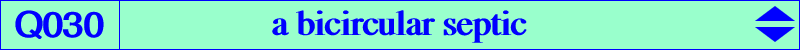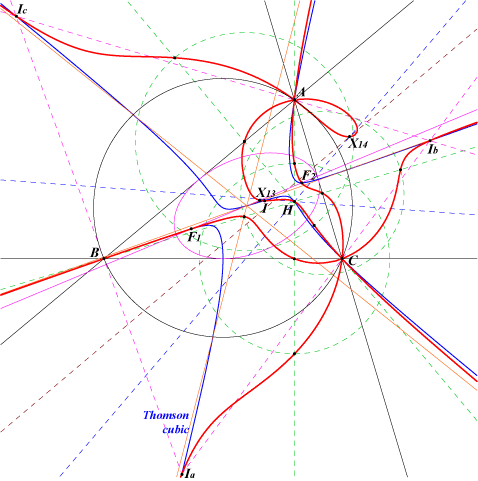too complicated to be written here. Click on the link to download a text file.X(1), X(4), X(13), X(14), X(74), X(80) excenters feet of the altitudes foci of the Steiner inscribed ellipse anti-points, see Table 77 points at infinity of the Thomson cubicLet ABC be a triangle and P a point. The line AP intersects the circumcircle of the triangle PBC at P and A'. Similarly define B', C'. A", B", C" are the projections of A', B', C' on the sidelines of ABC. The triangles ABC and A"B"C" are perspective for any P. Q030 is the locus of P such that these triangles are orthologic, together with the circumcircle of ABC (question raised by Antreas Hatzipolakis, Hyacinthos #10031). Q030 is a bicircular septic passing through A, B, C which are triple points. It has three real asymptotes parallel to those of the Thomson cubic. It meets the altitude AH at A (triple), H, its foot Ha on BC and two other points on the circle centered at Ha with radius sqrt(SB SC)/a. See also K001 = Neuberg cubic (locus property 12) and Q003 for other loci related to the same configuration. Another description of Q030 is the following : denote by Ab, Ac the projections of A on the lines PB, PC and by La the Euler line of the triangle AAbAc. Define Lb and Lc similarly. These three lines concur if and only if P lies at infinity or on Q030. In this case, the point of concurrence is the center of the rectangular circum-hyperbola through P and lies on the nine-point circle. (see the thread "Antreas' Feuerbach concurrence" at Hyacinthos, messages 10584 & sq.) One remarkable fact about Q030 is that it is invariant under antigonal conjugation. The isogonal transform of Q030 is Q037, an inversible bicircular quintic.